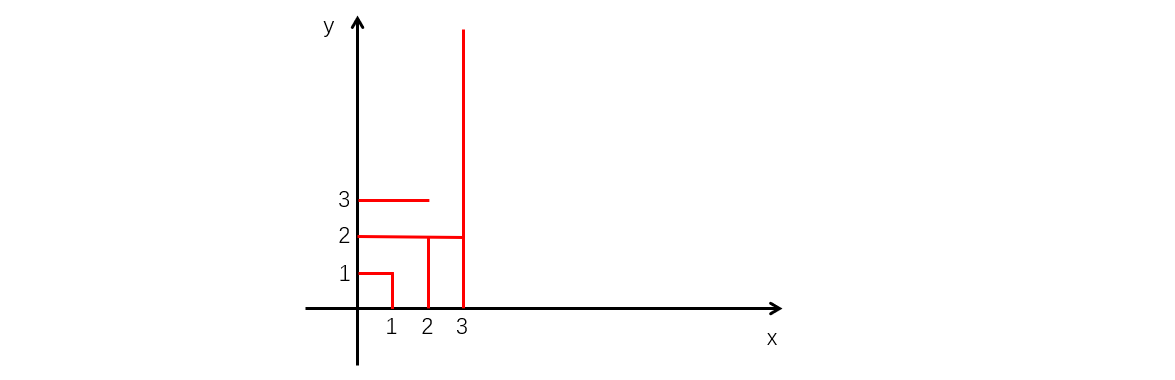# #770. 【UER #11】切割冰片

• 其中有 $m$ 个竖直向上的激光发射器，第 $i$ 个竖直向上的激光发射器位于 $(i,0)$，所发出的激光的强度为 $10^{100}$；
• 其中有 $n$ 个水平向右的激光发射器，第 $i$ 个水平向右的激光发射器位于 $(0,i)$，所发出的激光的强度为 $l_i$。### 样例一

#### input

3 3
1 4 2



#### output

11



### 样例二

#### input

10 10
7 8 5 8 3 2 3 4 4 6



#### output

2021



### 数据范围

$1$ $4$ $4$ $15$
$2$ $10$ $10$ $15$
$3$ $500$ $500$ $30$
$4$ $10^6$ $20$
$5$ $10^9$ $20$